# Optimization

You are currently browsing the archive for the Optimization category.

## Some Notes on Optimal Portfolio Theory

This note just reviews the derivation of portfolios that maximize the Sharpe ratio.

Suppose that you have some stocks that you want to invest in. We will think of the returns of these stocks as being a random column vector $G$ in $R^n$. Suppose that $r=E[G]\in R^n$ is a vector of the expected return of the stocks and $C= E\left[ (G-r) (G-r)^T\right]$ is the covariance matrix of $G$ with the superscript $T$ indicating the transpose, thus $C\in R^{n\times n}$.

We will often want to maximize the Sharp ratio of a portfolio which is defined as the expected return of the portfolio minus the risk free return divided by the standard deviation.  In order to simplify the math a little, we will assume that the risk free return is 0 and $C$ is positive definite, $a^T C a>0$ for all vectors $a\in R^n\setminus\{0\}$. Thus for our purposes, the Sharpe ratio for an “allocation vector” $a\in R^n\setminus\{0\}$ will be defined $$\rho(a) := \frac{E[a^T G]}{\sqrt{E[ (a^T G - a^T r)^2]}} = \frac{a^T r}{\sqrt{a^T C a}}.$$ We could say that the allocation vector is in dollars, so $a_1$ would be the dollar value of the stocks held in the portfolio for the first stock.  The value of $a_1$ could be negative indicating that the stock was shorted.

It is helpful to notice that the Sharpe ratio does not change if we double or triple the amount invested in each stock.  In fact, for any real number $\gamma\neq 0$ and any nonzero allocation vector $a\in R^n$, $$\rho(\gamma a)= \gamma \rho(a).$$ So, when maximizing $\rho$ we can restrict ourselves to vectors $a$ where $a^T C a=1$.

The matrix $C$ is real symmetric positive semidefinite, so it has a Cholesky decomposition $C=U^T U$ where $U$ is upper triangular.  Let $u= U a$.  Then $$1=a^T C a= a^T U^T U a = u^T u= ||u||^2,$$ so $u$ has norm 1. Thus if we want to maximize $\rho(a)$, it suffices (by restricting to vectors $a$ where $a^T C a=1$) to maximize $$\rho(a) = \frac{a^T r}{\sqrt{a^T C a}} = a^T r = u^T U^{-T} r$$ over all unit vectors $u$. (We use $U^{-T}$ to denote $(U^T)^{-1}$, the inverse transpose of $U$.)  The unit vector which maximizes $u^T U^{-T} r$ is simply $$u^*= \frac{U^{-T} r}{|| U^{-T} r||}.$$ We can now generate an optimal allocation vector $a^*$ by

$$a^* = U^{-1} u^*= \frac{U^{-1} U^{-T} r}{|| U^{-T} r||} = \frac{ (U^T U )^{-1} r}{|| U^{-T} r||} = \frac{ C^{-1} r}{|| U^{-T} r||}.$$ The scalar factor $|| U^{-T} r||$ has no effect on $\rho$, so $$a^{**} = C^{-1} r$$ is also an optimal allocation vector.  Note that the Sharpe ratio of $a^*$

$$\rho(a^{**})=\rho(a^*)=\frac{(a^{*})^T r}{\sqrt{(a^{*})^T C a^*}}=(a^{*})^T r= \frac{r^T U^{-1} U^{-T} r}{|| U^{-T} r||}= || U^{-T} r||.$$

### Example 1

Suppose that you want to invest in two uncorrelated stocks.  Assume that their expected returns are $r=( 0.001, 0.001)^T$ and their covariance matrix is $$C=\left(\begin{matrix} 10^{-4} & 0 \\ 0 & 10^{-4}\end{matrix}\right).$$  All optimal allocations $a$ of the stocks are multiples of $$a^{**} = C^{-1} r = \left(\begin{matrix} 10^{4} & 0 \\ 0 & 10^{4}\end{matrix}\right)( 0.001, 0.001)^T= (10, \ 10)^T.$$ This merely indicates that the optimal Sharpe ratio is attained if and only if you invest the same amount in money in each of these stocks.

### EXAMPLE 2

Suppose that you want to invest in two uncorrelated stocks.   Assume that their returns are $r=( 0.001, 0.0005)^T$ and their covariance matrix is $$C=\left(\begin{matrix} 10^{-4} & 0 \\ 0 & 10^{-4}\end{matrix}\right).$$  All optimal allocations $a$ of the stocks are multiples of $$a^{**} = C^{-1} r = \left(\begin{matrix} 10^{4} & 0 \\ 0 & 10^{4}\end{matrix}\right)( 0.001, 0.0005)^T= (10, \ 5)^T.$$ This indicates that the optimal Sharpe ratio is attained if and only if you invest the twice as much money in the first stock and a nonzero amount of money is invested.  Note that Kelly Criterion often indicates that your bets should be proportional to the edge of your investments, so it gives similar advice.

### EXAMPLE 3

Suppose that we have two gamblers.  The first gambler is willing to give you 2.2 times your wager if candidate A wins the election, but you lose the bet if candidate A does not win.  (I.e. if you wager $\$10$with the first gambler, the your net gain will be$\$22 – \$10 = \$12$ if you win.)   The second gambler is willing to pay you twice your bet if candidate B wins and you lose your bet with the second gambler if candidate B loses.

This could be called an arbitrage situation.

Let’s assume that there is a 50% chance that candidate A will win and a 50% chance that candidate B will win.  We can think of each gambler as being a stock that we can invest in.  The expected value of the first gambler is 0.1  (i.e. if you wager ${\$}10$with the first gambler, your expected net gain is 0.1*${\$}10$ = ${\$}1$.) The expected value of the second gambler is 0. The covariance matrix requires some computations. $$C_{11} = E[ (G_1-r_1)^2] = 1/2 ( (1.2 – 0.1)^2 + (-1 – 0.1)^2 ) = 1.21.$$ $$C_{12} = C_{21} = E[ (G_1-r_1)(G_2-r_2)] = 1/2 ( (1.2 – 0.1)(-1) + (-1 – 0.1)1 ) = -1.1.$$ $$C_{22} = C_{21} = E[ (G_2-r_2)^2] = 1/2 ( (-1)^2 + (1)^2 ) = 1.$$ $$C = \left(\begin{matrix} 1.21 & -1.1 \\ -1.1 & 1 \end{matrix}\right).$$ Interestingly,$C$is not invertible. This is because$(10, 11) C (10, 11)^T = 0$. This means that if you wager$\$10$ with gambler 1 and $\$11$with gambler 2, you will always win$\$1$.  If candidate A wins, then you gain $\$12$from the first gambler and lose$\$11$ to the second.  If candidate B wins, then you lose $\$10$to the first gambler and gain$\$11$ from the second.  Since you always win $\$1$, your volatility is zero and your Sharpe ratio is infinite. In the derivation, we assumed that$C$was positive definite, but in this example, it is not. In a future post, I would like to give a few more examples and maybe even compare the optimal Sharp ratio allocation with a Kelly allocation. ## Maximizing a function over integers (Part 2) In part 1, we defined the term strictly concave and introduced the theorem that if$f$is strictly concave and$f(x)=f(x+1)$, then the integer(s) that maximize$f$are exactly the set$\{\mathrm{floor}(x+1), \mathrm{ceil}(x)\}$which is usually a set containing only one number$\mathrm{round}(x+1/2)$. ### Parabolas The simplest concave functions are parabolas with the vertex at the top. All parabolas can be described by three real numbers$a$,$b$, and$c$, and the formula$y=a x^2 + b x + c$. For strictly concave parabolas$a<0$. For example, if$a=-2$,$b=3$, and$c=5$, then the function$y= f(x) = -2x^2 + 3 x +5$is the parabola shown below.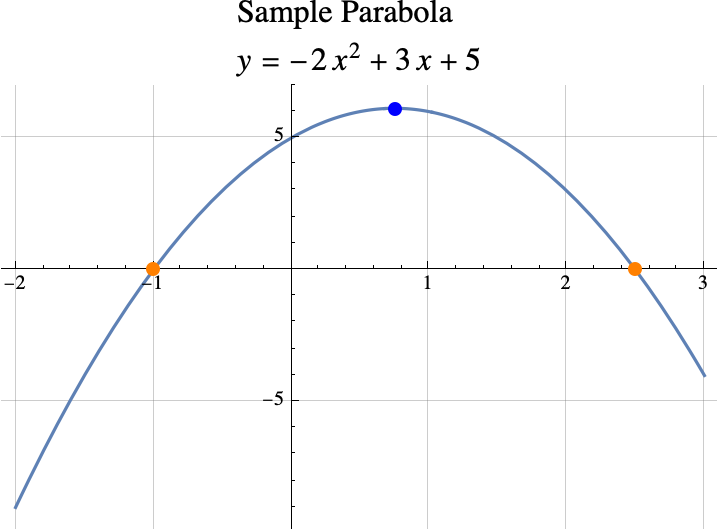If the vertex (shown above in blue) is above the x-axis, then the x-coordiante of the vertex can be computed by finding the points where the concave parabola crosses the x-axis (shown above in orange). In our case, the parabola$y=f(x)$crosses the x-axis when$0=y=f(x), or when \begin{aligned}0&= -2x^2 + 3 x +5\\0&=-(2x^2-3×-5)\\0&=-(2×-5)(x+1).\end{aligned}. If0=\alpha\cdot \beta$, then either$\alpha=0$or$\beta=0$. So, the parabola crosses the x-axis when either$0=2×-5$or$0=x+1$which means that it crosses when$x=5/2$or$x=-1$. Parabolas are symmetric about the vertical line going through the vertex, so the x-coordinate of the vertex is half way between the x-coordinates of the crossing points (a.k.a the roots of$f(x)). \begin{aligned} x_\mathrm{vertex} &= \frac{x_\mathrm{crossing1} + x_\mathrm{crossing2}}2\\&= \frac{ -1 +5/2}2\\&=\frac{3/2}2\\&=3/4.\end{aligned} So the x-coordinate of the vertex isx=3/4$. This is also the real number that maximizes$f(w)$over all real numbers$w$. In general, the x-coordinates of the crossing points can be found with the quadratic formula $$x=\frac{-b\pm\sqrt{b^2-4ac}}{2 a}.$$ The$\pm$sign means that one crossing can be found by replacing$\pm$with + and the other can be found by replacing the$\pm$with -. But, if you have one number that is$x_1=\alpha+\beta$and another that is$x_2=\alpha-\beta$, then the average of the two numbers is just$\alpha$. In other words if the two values of$x$are$x=\alpha\pm\beta$, then the average value is just$\alpha$. So, to computer the average x-coordinate of the crossing points, all we have to do is remove the$\pm$sign and whatever it is applied to from the quadratic formula. $$x_\mathrm{vertex} = \frac{-b}{2 a}.$$ ### theorem #2 Informally, if$y=f(x)$is a parabola,$f(x) = a x^2 + b x + c$, and$a<0$, then the integer(s) that maximize$f(x)$are the set$\{\mathrm{floor}(x+1/2), \mathrm{ceil}(x-1/2)\}$where$x=\frac{-b}{2 a}$. If$x$is an integer plus 1/2 (e.g. 2.5, 7.5, …), this set has two elements$x+1/2$and$x-1/2$. If$x$is not an integer plus 1/2, the set has only one element$\mathrm{round}(x)$and that is the integer that produces the highest possible value of$f(z)$among all integers$z$. ### examples Example 1: If$f(x)= -2x^2 + 3 x +5$, then$a=-2$,$b=3$, and$c=5$. The values of$x$in the theorem is the same as the x-coordinate of the vertex $$x=\frac{-b}{2a} = \frac{-3}{2\cdot(-2)}= \frac{-3}{-4}=3/4.$$ The integer(s) that maximize$f(z)$among all integers$zare the set \begin{aligned}\{\mathrm{floor}(x+1/2), \mathrm{ceil}(x-1/2)\}&= \{\mathrm{floor}(3/4+1/2), \mathrm{ceil}(3/4-1/2)\}\\&= \{\mathrm{floor}(5/4), \mathrm{ceil}(1/4)\}\\&=\{1\}.\end{aligned} Example 2: If we lower the parabola from example 1 by a little bit settingf(x)= -2x^2 + 3 x +\sqrt{17}$, then$a=-2$,$b=3$, and$c=\sqrt{17}$. The value of$x$in the theorem is the same as the x-coordinate of the vertex$x=\frac{-b}{2a} =3/4.$The result does not depend on$c$, so as in example 1, the integer that maximizes$f(z)$among all integers$z$is$z=\mathrm{round}(3/4)=1$. Example 3:$f(x)= -x^2+x = (1-x)x$, then$a=-1$,$b=1$, and$c=0$. The value of$x$in the theorem is the same as the x-coordinate of the vertex $$x=\frac{-b}{2a} =\frac{-1}{2\cdot (-1)}=1/2.$$ The integer(s) that maximize$f(z)$among all integers$zare the set \begin{aligned}\{\mathrm{floor}(x+1/2), \mathrm{ceil}(x-1/2)\}&= \{\mathrm{floor}(1/2+1/2), \mathrm{ceil}(1/2-1/2)\}\\&= \{\mathrm{floor}(1), \mathrm{ceil}(0)\}\\&=\{1,0\}.\end{aligned} The integers that maximizef(z)$among all integers$z$are 0 and 1. If we look at the graph of$f(x)$below, we can see that the graph crosses the x-axis at$x=0$and$x=1$. So,$f(0)=f(1)=0$. All other integers inputs produce negative values.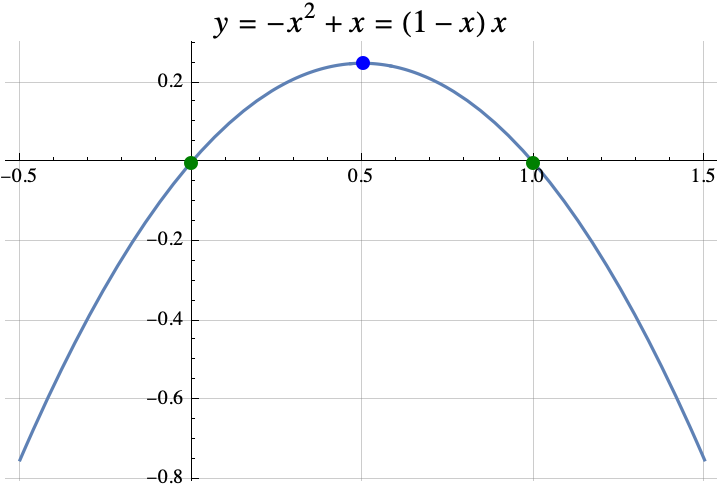### the spirit of the proof It turns out that Theorem 2 can be proven from theorem 1. Recall that in Theorem 1, we wanted to find the value of$x$where$f(x)=f(x+1)$. If we found that value, then the integer(s) that maximize$f$are exactly the set$\{\mathrm{floor}(x+1), \mathrm{ceil}(x)\}$. In Theorem 2,$f(x) = a x^2 + b x + 1$. So if$f(x)=f(x+1), then \begin{aligned}a x^2 + b x + c &= a (x+1)^2 + b (x+1) + c\\a x^2 + b x &= a (x+1)^2 + b (x+1)\\ a x^2 + b x &= a (x^2+2x+1)+ b x+b\\ a x^2 &= a x^2+2ax+a+ b \\ 0 &= 2ax+a+ b \\ -a-b &= 2ax \\ \frac{-a-b}{2a} &= x\\ \frac{-b}{2a}-\frac12 &= x\\\end{aligned}. Thus the integers that maximizef(x)must be \begin{aligned} \{\mathrm{floor}(x+1), \mathrm{ceil}(x)\ \}&= \{\mathrm{floor}(\frac{-b}{2a}-\frac12+1), \mathrm{ceil}(\frac{-b}{2a}-\frac12)\} \\ &= \{\mathrm{floor}(\frac{-b}{2a}+\frac12), \mathrm{ceil}(\frac{-b}{2a}-\frac12)\}.\end{aligned} ### Why DId I WANTED to know this I was looking at several games where the optimal strategy depended on finding an integert$that maximized$f(t)$. In the game Slay the Spire, I wanted to maximize the amount of poison damage that I was going to do with the cards “Noxious Fumes” and “Catalyst”. If I played the “Catalyst” on turn$t$and the combat ended on turn$T$, then the poison damage done was $$\frac{(t+1)t}{2} -1 + (T-t)t$$ where$ \frac{(t+1)t}{2}-1$(note the triangle number) was the damage done by “Noxious Fumes” and$ (T-t)t$was the additional damage done by playing the Catalyst. I wanted to maximize$f(t) = (T-t)t = -t^2 + T t$. Using Theorem 2,$a=-1$,$b=T$, and$c=0$. The Theorem says that the maximum damage occurs when you play the “Catalyst” on round$t$where$t$is contained in the set$\{\mathrm{floor}(x+1/2), \mathrm{ceil}(x-1/2)\}$with$x=\frac{-b}{2 a}$. So$x=\frac{-T}{2\cdot(-1)}=T/2$. The best time to play the Catalyst was around half way through the combat. The catalyst should be played on round$T/2$if$T$is even. If$T$is odd, then the best round to play the “Catalyst” was for$t$in the set $$\{\mathrm{floor}(T/2+1/2), \mathrm{ceil}(T/2-1/2)\}= \{\frac{T+1}{2}, \frac{T-1}{2} \}.$$ ### summary So the first two theorems formalized two rules of thumb: 1. If you can find an$x$where$f(x)=f(x+1)$, then the optimal integer(s) is$z=\mathrm{round}(x+1/2)$with a special round that provides two answers if$x$is an integer, and 2. If$f(x)=a x^2 + b x + c$(a parabola), then the optimal integer(s) is$z=\mathrm{round}(x)$where$x=\frac{-b}{2 a}$which is the$x$that maximizes$f(w)$over all real numbers$w$(i.e. the x-coordinate of the vertex). If you want to see a more formal mathematical writeup, click here. ## Maximizing a function over integers (Part 1) I proved a few simple but interesting theorems about integers that maximize a function$f$when$f$is a strictly concave function that maps real numbers to real numbers. For example, the real number that maximizes$f(x)=x(1-x)$is$x=1/2$, but among all the integers, the maximum possible value of the function is 0. And that maximum is achieved twice with integers 0 and 1,$f(0)=0=f(1)$.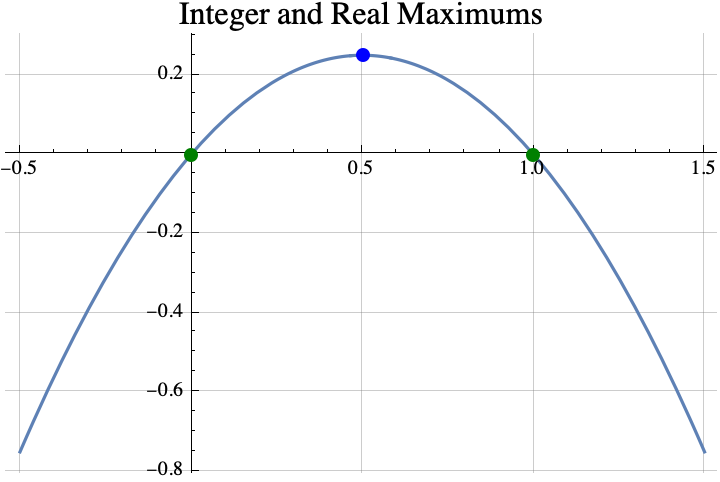A function is strictly concave if you can pick any two points on its graph, draw a line between them, and the curve$y=f(x)$between the two points lies entirely above the line between the two points.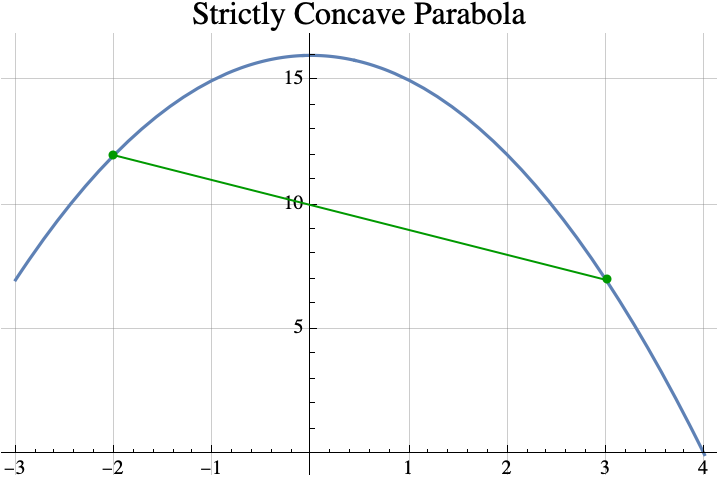### Theorem #1 Informally stated, the first theorem is that if you can find a real number$x$such that$f(x)=f(x+1)$, then the integer(s) that maximize the concave function$f$are the set$\{\mathrm{floor}(x+1), \mathrm{ceil}(x)\}$where “floor” just means round down and “ceil” means round up. That set usually contains only one element which is$\mathrm{round}(x+1/2)$, but if$x$is an integer, then it will contain two consecutive integers$x$and$x+1$. For example, if$f(x) = 4-4x^2$, then the value of$x$that satisfies$f(x)=f(x+1)$is$x=-1/2$because$f(-1/2)=3=f(1/2)$. So the theorem says that any integer that maximizes$fmust be in the set \begin{aligned}\{\mathrm{floor}(x+1), \mathrm{ceil}(x)\} &= \{\mathrm{floor}(-1/2+1), \mathrm{ceil}(-1/2)\}\\&= \{\mathrm{floor}(1/2), \mathrm{ceil}(-1/2)\} \\&= \{0\}.\end{aligned} The integer that does the best is 0 which is also the real number that maximizesf$.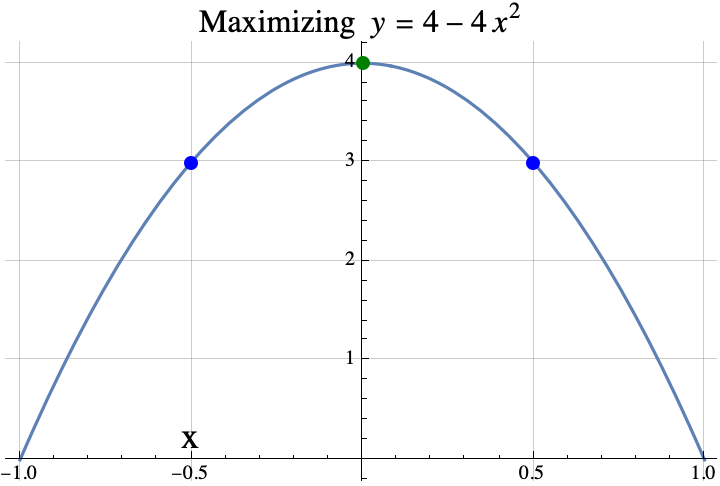Here is another example. If$f(x) = \sin(x)$, then the value of$x$that satisfies$f(x)=f(x+1)$is$x=1.0708$because$f(1.0708)=0.877583=f(2.0708)$. So the theorem says that any integer that maximizes$fmust be in the set \begin{aligned}\{\mathrm{floor}(x+1), \mathrm{ceil}(x)\} &= \{\mathrm{floor}(1.0708+1), \mathrm{ceil}(1.0708)\}\\&= \{\mathrm{floor}(2.0708), \mathrm{ceil}(1.0708)\} \\&= \{2\}.\end{aligned}. The integer that does the best is 2.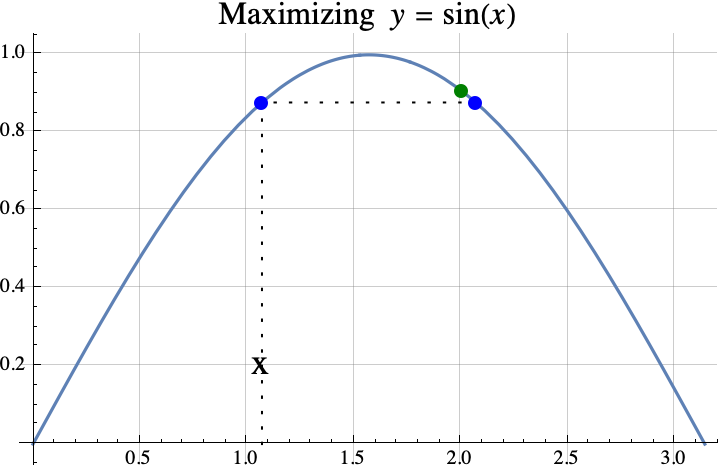So, that was the first theorem. In part 2, we state a second theorem about finding the integer which maximizes a quadratic with some examples and one application the game “Slay the Spire”. If you want to see a more formal mathematical writeup, click here. ## Richardson Extrapolation Dr Xu at Penn State introduced me to Richardson Extrapolation way back in the early 90’s. We used it to increase the speed of convergence of finite element analysis algorithms for partial differential equations, but it can be used for many numerical methods. https://en.wikipedia.org/wiki/Richardson_extrapolation ## “Multi-stage Markov Chain Monte Carlo Methods for Porous Media Flows” I was doing a little bit of research on Mulit-stage Markov Chains for a friend when I ran across this nice set of slides on Multi-stage Markov Chains for diffusion through porous media (i.e. oil or water flowing through the ground) by Pereira and Rahunanthan. The authors first review the multi-scale equations for diffusion under pressure (see Darcy’s law) and mixed finite element analysis. Then, interestingly they introduce a Bayesian Markov chain Monte Carlo method (MCMC) to get approximate solutions to the differential equation. They use Multi-Stage Hastings-Metropolis and prefetching (to parallelize the computation) to improve the speed of MCMC. The slides also display the results of their algorithm applied to aquifer contamination and oil recovery. It’s really cool how methods used for probabilistic graphical models can be used to approximate the solutions to partial differential equations. I’m hoping to get back to 2048 in a few weeks. Turns out that it takes a long time to write code, make the code look nice, run the code, analyze the results, and then put together a blog post. It’s much easier and quicker to read papers and summarize them or to try to explain things you already know. Have a great weekend. – Hein ## “Randomized Numerical Linear Algebra (RandNLA): Theory and Practice” Nuit Blanche has a nice summary of the FOCS 2012 53rd Annual IEEE Symposium on Foundations of Computer Science Workshop on “Randomized Numerical Linear Algebra (RandNLA): Theory and Practice“. Faster random algorithms for QR, SVD, Eigenvalues, Least Squares, … using random projections and other techniques were discussed. ## “Stochastic Superoptimization” and “Programming by Optimization” John Regehr writes this post about a compiler speed optimization technique called “Stochastic Superopimization“. “Stochastic Superopimization” systematically searches for algorithmic improvement in code using machine learning algorithms similar to multi-armed bandit strategies. It appears to be related to “Programming by Optimization“. “Stochastic Superopimization” is more like a very good optimization flag on a compiler. “Programming by Optimization” is constructing the program in such a fashion that design options are exposed and easily manipulable by an optimization program trying to maximize some performance metric. The “Programming by Optimization” community seems to mostly use BOA, the Bayesian optimization algorithm (see , ). I am hoping to read and write more about both of these ideas later. ## “Algorithm Portfolio Design: Theory vs. Practice” In “Algorithm Portfolio Design: Theory vs. Practice“, Gomes and Selman (2013) study the use of a portfolio of stochastic search algorithm to solve computationally hard search problems. Here are some interesting quotes from the paper: “Our studies reveal that in many cases the performance of a single algorithm dominates all others, on the problem class under consideration.” “Given the diversity in performance profiles among algorithms, various approaches have been developed to combine different algorithms to take into account the computational resource constraints and to optimize the overall performance. These considerations led to the development of anytime algorithms (Dean and Boddy 1988), decision theoretic metareasoning and related approaches (Horvitz and Zilberstein 1996; Russell and Norvig 1995), and algorithm portfolio design (Huberman et al. 1997).” “In addition, we also show that a good strategy for designing a portfolio is to combine many short runs of the same algorithm. The effectiveness of such portfolios explains the common practice of “restarts” for stochastic procedures, where the same algorithm is run repeatedly with different initial seeds for the random number generator. (For related work on the effectiveness of restarts, see e.g., Aldous and Vazirani 1994; Ertel 1991; Selman and Kirkpatrick 1996.)” ## “Trustworthy Online Controlled Experiments: Five Puzzling Outcomes Explained” “…we often joke that our job, as the team that builds the experimentation platform, is to tell our clients that their new baby is ugly, …” Andrew Gelman at Statistical Modeling, Causal Inference, and Social Science pointed me towards the paper “Trustworthy Online Controlled Experiments: Five Puzzling Outcomes Explained” by Ron Kohavi, Alex Deng, Brian Frasca, Roger Longbotham, Toby Walker, and Ya Xu all of whom seem to be affiliated with Microsoft. The paper itself recounted five online statistical experiments mostly done at Microsoft that had informative counter-intuitive results: • Overall Evaluation Criteria for Bing • Click Tracking • Initial Effects • Experiment Length • Carry Over Effects. The main lessons learned were: • Be careful what you wish for. – Short term effects may be diametrically opposed to long-term effects. Specifically, a high number clicks or queries per session could be indicative of a bug rather than success. It’s important to choose the right metric. The authors ended up focusing on “sessions per user” as a metric as opposed to “queries per month” partly due to a bug which increased (in the short-term) queries and revenues while degrading the user’s experience. • Initial results are strongly affected by “Primacy and Novelty”. – In the beginning, experienced users may click on a new option just because it is new, not because it’s good. On the other hand, experienced users may be initially slowed by a new format even if the new format is “better”. • If reality is constantly changing, the experiment length may not improve the accuracy of the experiment. The underlying behavior of the users may change every month. A short-term experiment may only capture a short-term behavior. Rather than running the experiment for years, the best option may be to run several short-term experiments and adapt the website to the changing behavior as soon as the new behavior is observed. • If the same user is presented with the same experiment repeatedly, her reaction to the experiment is a function of the number of times she has been exposed to the experiment. This effect must be considered when interpreting experimental results. • The Poisson Distribution should not be used to model clicks. They preferred Negative Binomial. The paper is easy to read, well written, and rather informative. It is especially good for web analytics and for anyone new to experimental statistics. I found the references below to be especially interesting: ## “Linear Bandits in High Dimension and Recommendation Systems” Thanks to Nuit Blanche for pointing me towards the presentation by Andrea MontanariCollaborative Filtering: Models and Algorithms” and the associated Deshpande and Montanari paper “Linear Bandits in High Dimension and Recommendation Systems” (2012). In the presentation, Montanari reviews Spectral, Gradient Descent, Stochasitc Gradient Descent, Convex Relaxation, and Linear Bandit methods for approximating the standard linear model for recommendation systems and some accuracy guarantees. Assuming thej$th movie has features$v_{j1}, v_{j2}, \ldots, v_{jr}$, then the$i$th viewer gives the rating$R_{ij} = \langle u_i, v_j \rangle +\epsilon_{ij}$where$u_i$is an$r$dimensional vector representing the preferences of$i$th viewer and$\epsilon_{ij}\$ is Gaussian noise.

The paper introduces a new Linear Bandit method,  Smooth Explore, better suited for recommendation systems.  Their method is motivated by the three objectives:

• Constant-optimal cumulative reward,
• Constant-optimal regret, and
• Approximate monotonicity (rewards approximately increase with time).

Smooth Explore estimates the user preferences vectors with a regularized least squares regression.  Proofs of optimality and numerical results are provided.

« Older entries